/
Features

# Tessellation of 4x4 Bezier Patches for the Intel Pentium III Processor

In the latest installment of Optimizations Corner, Haim Barad takes a look at optimizing content based upon parametric surfaces. With the help of Ronen Zohar and a floating teapot demo, Barad demonstrates 4x4 Bezier patches and how, by creating an optimized engine that incorporates tessellated surfaces, one can exploit features such as continuous LOD and non-rigid body deformation.

This month, we take a look at optimizing content based upon parametric surfaces. To do this we concentrate on a specific case, 4x4 Bezier patches. By creating an optimized engine that incorporates tessellated surfaces, we can exploit features such as continuous LOD and non-rigid body deformation. This article features the work of Ronen Zohar, a member of Intel Israel's Media Team, who has experience with a wide range of 3D engine optimizations under his belt, including Intel's Processor Specific Graphics Pipeline for Direct3D since release 6.

With increased processor power, previous offline 3D tasks, such as dealing with high-order surfaces, can now be performed at run-time. A big advantage of high-order surfaces is that their relatively small data set makes them perfect for bandwidth-limited Internet applications. Since current 3D HW can render triangles only, we must generate triangles from the high-order surface using a process called “tessellation.” In this article, we show how to perform uniform tessellation of 4x4 Bezier surfaces (or patches), using the SIMD power of the Pentium III processor’s Streaming SIMD Extensions. Our implementation is written in C++ using the Streaming SIMD Extensions support classes and the Intel C/C++ compiler.

Introduction to Bezier Curves and Surfaces

Bezier curves are parametric curves defined by a set of n+1 control points (P0-Pn), which define the “basic shape” of the curve, and a parameter (t), in the range of [0..1]. The mathematical representation of a Bezier curve is: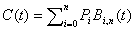where the polynomials, Bi,n, (called Bezier basis functions) are defined as: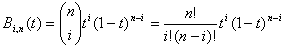Bezier curves are polynomials of n-th degree that interpolate between the control points, where C(0) = P0 and C(1) = Pn.

Bezier surfaces are two-parameter expansions to the Bezier curve concept. Bezier surfaces are defined by an (n+1) x (m+1) matrix of control points (P), which define the “basic shape” of the surface, and two parameters (s and t), both in the range [0..1]. The mathematical representation of a Bezier surface is: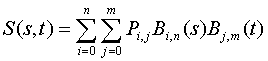Lighting and reflection mapping calculations require the normal vector for each surface point (s, t). To calculate this vector, we first differentiate the surface equation with respect to s and t. The resultant partial derivatives define two vectors in the plane tangent to the surface at (s, t). The normal vector for the surface at this point is the cross product of these two tangent vectors: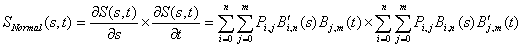In this paper, we deal with 4x4 Bezier curves, which are bi-cubic polynomials. The basis functions and their derivatives for the n=3 case are: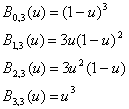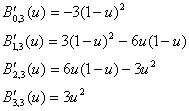The Tessellation Process

There are three basic steps in the tessellation process: taking samples of the parametric surface, connecting these samples into triangles, and generating the tessellated surface vertices.

Many techniques and algorithms deal with the problem of sampling the parametric surface. Some are based on the surface curvature (the smaller the surface curvature, the fewer triangles you need to approximate the surface shape). We use the simplest algorithm, which uniformly divides the [0…1]2 (s, t) plane into some tessellation level , and then samples the surface at these points. Note that we designed our data structures so that any other tessellation-sampling algorithm can be used instead of our simple algorithm.

After determining the sample points, we precalculate the basis function values at these sample points and generate an indices list that accompanies the tessellation data. This indices list describes the triangle connectivity of the surface sample points that will be generated by the tessellation process. The indices list will be submitted to the rasterizer, along with the surface sample points, in order to render the tessellated surface.

We can use two methods to generate the tessellated surface vertices. The simplest method is to compute the surface position and normal in object space (the space where the control points are defined), and then feed these vertices to the triangle transform and lighting routines. A more complex, but faster, method is to transform the control points to screen space and tessellate using the transformed control points. In our case, we will need 16 transformations, regardless of the number of vertices generated by the tessellation process.  The two drawbacks of the second method are:

1. For small vertex counts, it is cheaper to transform only an already tessellated surface.
2. Lighting calculations sometimes require object or world space position. In this case, we will have to tessellate once for screen space and once for object/world space.

We’ve implemented only the second method, but the implementation of the first method can be done easily by commenting the transformation and lighting calculations from the routines.

Data Structures and Classes

The CBezierTessellation Class:

The CBezierTessellation class serves two purposes. The first is to hold the triangle indices data for the current tessellation level. This data is sent to the rasterization HW and indicates how to connect the vertices generated by the tessellation process into triangles. The second purpose is to hold precalculated values for the basis functions and their derivatives for the chosen (s, t) parameter pair at each sample point. Since our tessellation is not dependent on the position of the control points of the Bezier patch, we can use one CBezierTessellation object for all the Bezier patches in our application.

The CBezierSurface and CBezierPatch Classes:

A single, 4x4 patch can generate only a limited set of objects. This is why we often see sets of 4x4 patches connected to each other to produce the object, where each patch defines only a small portion of the object’s surface. Neighboring patches are connected by sharing the control points along the common edge of the connected surface. To use the data efficiently, we define two classes: CBezierSurface, which holds all the control points used by the object, and CBezierPatch, which holds a 4x4 matrix of indices to control points stored in the patch parent CBezierSurface object.

Streaming SIMD Extensions Implementation

Setup

We use Streaming SIMD Extensions to evaluate the surface position and surface normal for four sample points in parallel. Within the data structure, the basis function values for the sample points (s, t) are organized into groups of four values each. To improve cache locality, we store these values in continuos memory blocks. The memory footprint of the basis function values looks like:

B0,3(s0),B0,3(s1),B0,3(s2),B0,3(s3),B1,3(s0),B1,3(s1),,,,B3,3(t0), B3,3(t1), B3,3(t2), B3,3(t3)

For every iteration of four sample points, we need 32 floating-point values (4 s values x 4 basis functions + 4 t values x 4 basis functions), or 4 cache lines. We use the prefetch instruction to tell the processor that the next 4 cache lines will be used in the next iteration. This way, no cache misses occur during the execution of the tessellation algorithm.

We also setup the tessellation process by expanding the control points four times. We need to expand  the current set of control points because the algorithm uses the control points values in parallel to generate four vertices. (When tessellating directly to screen space,  we need to expand the control points after the transformation to screen).

Position calculation

There is a big difference between the tessellation of screen space surfaces and the tessellation of object space surfaces. For object space surfaces, we only need to calculate the surface position “by the book”. For screen space surfaces, we usually need to transform using a perspective projection. The perspective projection changes the actual position of the x, y coordinates based on their z-value. To make the surface persistent in projective transformations, we must convert our patch to a “Rational Bezier surface”. A Rational Bezier surface is based on a set of homogenous control points coming from the control-point transformation algorithm. The fourth coordinate (W) is used as a weight that divides all the other control points coordinates: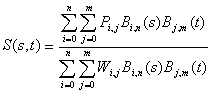The last stage of the surface position generation is the reformatting of the output data to the rasterizer. The data comes out as groups of 4 X’s, 4 Y’s, 4 Z’s and 4 W’s, while the rasterizer expects x, y, z, w vectors. We use the _MM_TRANSPOSE4_PS macro to transpose the values to the correct format.

Finally, the code for the tessellation of surface position is:

// Zero the vertex coordinates
vertex.x = vertex.y = vertex.z = vertex.w = _ZERO_;
for (k=0;k<4;k++) {
for (t=0;t<4;t++) {
// pre-multiply basis function values for s & t
F32vec4 coeff = coeffs[k] * coeffs[t + 8];
// get the control point based on its index
DWORD idx = _indices[t][k];                                                 PIIIVector4 *pt = &projBase[idx];
// sum the vertex components
vertex.x += coeff*pt->x;
vertex.y += coeff*pt->y;
vertex.z += coeff*pt->z;
// Weight calculation- required only when tessellating directly
// to screen space
vertex.w += coeff*pt->w;
}
}

// Perspective division - required only when tessellating
//directly to screen space
F32vec4 rhw = rcp(vertex.w);

// 1 over w using rcp
vertex.x *= rhw;
vertex.y *= rhw;
vertex.z *= rhw;

// Transpose position values from [xxxx], [yyyy], [zzzz], [wwww]
// to [x,y,z,w]X4 format.
_MM_TRANSPOSE4_PS(vertex.x, vertex.y, vertex.z,rhw);

// Store the four vertices position, using unaligned move
storeu(&vtx.sx, vertex.x);
storeu(&vtx.sx, vertex.y);
storeu(&vtx.sx, vertex.z);
storeu(&vtx.sx,rhw);

Surface Normal Calculation

To calculate the surface normals, we must first calculate the two tangent vectors to the surface. We calculate the s tangent vector by replacing the s-based basis function value in the position calculation with the value of its derivative at the sample point s. Similarly, we calculate the t tangent vector by replacing the t-based basis function value with the value of its derivative at the sample point t. The cross product of these vectors generates the surface normal at the sampling point. To do this calculation efficiently, we precalculate all the basis function derivatives data needed during the process, and store the derivative values together with the basis function values that are already stored for the position calculation.  The code for this calculation is:

normk.x = normk.y = normk.z = normt.x = normt.y = normt.z = _ZERO_;
for (k=0;k<4;k++) {
for (t=0;t<4;t++) {
PIIIVector3 *wpt = &worldBase[idx];
coeff = coeffs[k+4] * coeffs[t + 8];
normt.x += coeff * wpt->x;
normt.y += coeff * wpt->y;
normt.z += coeff * wpt->z;
coeff = coeffs[k] * coeffs[t + 12];
normk.x += coeff * wpt->x;                                                normk.y += coeff * wpt->y;
normk.z += coeff * wpt->z;
}
}

PIIIVector3 norm;

// Cross product of partial derivatives
norm.x = normk.y*normt.z - normk.z*normt.y;
norm.y = normk.z*normt.x - normk.x*normt.z;
norm.z = normk.x*normt.y - normk.y*normt.x;

// One over size of normal
F32vec4 size = rsqrt(norm.x*norm.x + norm.y*norm.y + orm.z*norm.z);

// Normalize the surface normal
norm.x *= size;
norm.y *= size;
norm.z *= size;

Performance

These performance numbers are measured on the “Utah teapot” Bezier surface, which is made from 306 control points and 32, 4x4 Bezier patches. The numbers are measured in Kcycles for the tessellation of the complete teapot on Pentium III processor @ 500 Mhz.

 Tessellate position only Tessellation level # of Triangles Legacy code Pentium III code Ratio 2 64 1280 1150 111% 3 256 2400 1550 155% 4 576 3880 1770 219% 6 1600 6500 2800 232% 8 3136 8570 4050 212% 12 7744 11500 6800 169% 18 18496 13500 9650 140% 24 33856 14500 11700 124%

 Tessellate position and calculate one directional light in world space Tessellation level #of triangles Legacy code Pentium III code Ratio 2 64 2800 1610 174% 3 256 5100 2770 184% 4 576 7100 3300 215% 6 1600 10100 5380 188% 8 3136 12000 7370 163% 12 7744 13800 10450 132% 18 18496 14850 12850 116% 24 33856 15530 13880 112%

Ideas for Future Improvements

The normal calculation and, in point light cases, the extra tessellation to object/world coordinates, is very compute intensive. There are several ways to approximate the surface normals using different levels of interpolations of the surface edges. In our code, we implemented a simple linear interpolation. Other interpolation techniques use Hermite basis functions, which are based on the second-derivative values on the surface edges. All these techniques produce a fairly decent image, as long as the surface curvature is relatively small.

Bezier patches can save communications bandwidth, as well as add to the photo-realism of the application. The optimization techniques shown enables real-time Bezier patch tessellation within a 3D engine; thus encouraging this type of content to be more common in real time applications.

A demo utilizing the techniques discussed in this article can be found here.

Haim Barad has a Ph.D. in Electrical Engineering (1987) from the University of Southern California. His areas of concentration are in 3D graphics, video and image processing. Haim was on the Electrical Engineering faculty at Tulane University before joining Intel in 1995. Haim is a staff engineer and currently leads the Media Team at Intel's Israel Design Center (IDC) in Haifa, Israel.

### Latest Jobs

#### Treyarch

5.8.23
Producer

Remote (United States)
5.18.23
Senior Gameplay Engineer

#### University of Canterbury

Christchurch, Canterbury, New Zealand
5.17.23
Academic in Game Arts and Animation

#### Fred Rogers Productions

Hybrid (424 South 27th Street, Pittsburgh, PA, USA
5.19.23
Producer - Games & Websites
More Jobs

#### Game Developer Job Board

Browse open positions across the game industry or recruit new talent for your studio